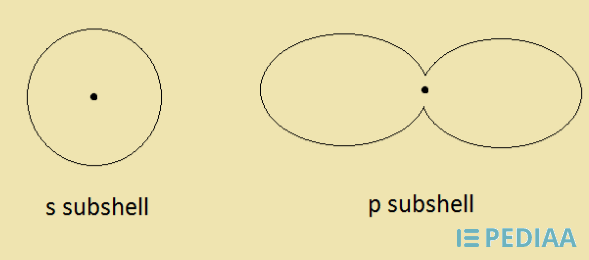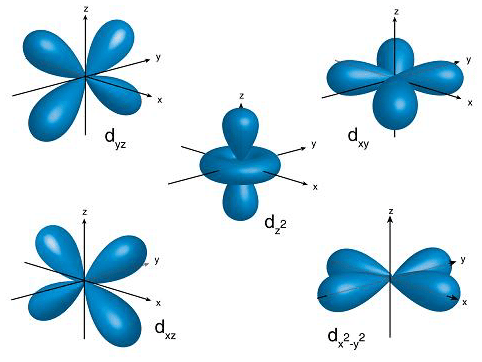# Difference Between Shell Subshell and Orbital

## Main Difference – Shell vs Subshell vs Orbital

Atom is the basic unit that composes matter. In the past, scientists believed that atoms could not be divided further. But later discoveries revealed information about subatomic particles, which indicated that atoms could be further divided into subatomic particles. The three main subatomic particles are electrons, protons, and neutrons. Protons and neutrons together make the nucleus, which is the central core of the atom. Electrons are in continuous movement around this nucleus. We cannot determine the exact location of an electron; however, electrons move in certain pathways. The terms shell, subshell and orbital refer to the most probable pathways that an electron can move. The main difference between shell subshell and orbital is that shells are composed of electrons that share the same principal quantum number and subshells are composed of electrons that share the same angular momentum quantum number whereas orbitals are composed of electrons that are in the same energy level but have different spins.

### Key Areas Covered

1. What is a Shell

– Definition, Structure, and Properties
2. What is a Subshell

– Definition, Structure, and Properties
3. What is an Orbital

– Definition, Structure, and Properties
4. What is the Difference Between Shell Subshell and Orbital

– Comparison of Key Differences

Key Terms: Atom, Electrons, Orbital, Quantum Number, Shell, Subshell## What is a Shell

A shell is the pathway followed by electrons around an atom’s nucleus. These are also called energy levels since these shells are arranged around the nucleus according to the energy that an electron in that shell is composed of. The shell that has the lowest energy is nearest to the nucleus. The next energy level is located beyond that shell.

In order to recognize these shells, they are named as K, L, M, N, etc. The shell in the lowest energy level is K shell. But, scientists have named these shells using quantum numbers. Each and every shell has its own quantum number. The quantum number given for the shells is named as the principal quantum number. Then the shell at the lowest energy level is n=1.

All shells do not hold the same number of electrons. The lowest energy level can only hold a maximum of 2 electrons. The next energy level can hold up to 8 electrons. There is a pattern of the number of electrons that a shell can hold. This pattern is given below.

 Principal Quantum Number (n) Maximum Number of Electrons n=1 2 n=2 8 n=3 18 n=4 32 n=5 32 n=6 32

Therefore, the maximum number of electrons that any shell can hold is 32. No shell can have more than 32 electrons. Higher shells can hold more electrons than that of lower shells.

The presence of these shells indicates that the energy of an atom is quantized. In other words, there are discrete energy values for the electrons that are in the movement around the nucleus.Figure 1: Atomic Shells

The electrons in these shells can be transferred from one shell to another by either absorbing or releasing energy. The amount of energy that is being absorbed or released should be equal to the energy difference between two shells. If not, this transition would not occur.

## What is a Subshell

A subshell is the area in which electron moves within a shell. These are named according to the angular momentum quantum number. There are 4 major types of subshells that can be found in a shell. They are named as s, p, d, f. Each subshell is composed of several orbitals. The number of orbitals that are in subshells is given below.

 Subshell Number of Orbitals Maximum Number of Electrons s 1 2 p 3 6 d 5 10 f 7 14

These subshells are also arranged according to the energy that they are composed of. At lower shells, the ascending order of the energy of subshells is as s<p<d<f. But at higher shells, this order will be different.Figure 02: Shapes of Subshells

These subshells have a unique 3D structure. s subshell is spherical. p subshell is dumbbell shaped. These shapes are given above.

## What is an Orbital

Orbital is a mathematical function that describes the wave-like behavior of an electron. In other words, the term orbital explains the exact movement of an electron. A subshell is composed of orbitals. The number of orbitals that a subshell has depends on the subshell. This means the number of orbitals present in a subshell is a unique feature for a subshell.

 Subshell Number of Orbitals s 1 p 3 d 5 f 10

However, one orbital can hold only a maximum of two electrons. These electrons are in the same energy level, but different from each other according to their spin. They always have opposite spins. When electrons are filled into the orbitals, they are filled according to the Hund’s Rule. This rule indicates that every orbital in a subshell is singly occupied with electrons before any orbital is doubly coupled.Figure 3: Shapes of d Orbitals

The above image shows the shapes of d orbitals. Since one d subshell is composed of 5 orbitals, the above image shows the 5 different shapes of these orbitals.

## Difference Between Shell Subshell and Orbital

### Definition

Shell: Shell is the pathway followed by electrons around an atom’s nucleus.

Subshell: Subshell is the pathway in which an electron moves within a shell.

Orbital: Orbital is a mathematical function that describes the wave-like behavior of an electron.

### Name of the Quantum Number

Shell: A shell is given the principal quantum number.

Subshell: A subshell is given the angular momentum quantum number.

Orbital: An orbital is given the magnetic quantum number.

### Maximum Number of Electrons

Shell: A shell can hold up to a maximum of 32 electrons.

Subshell: The maximum number of electrons that a subshell can hold depends on the type of subshell.

Orbital:  The maximum number of electrons that an orbital can hold is 2.

### Conclusion

An atom is composed of electrons, protons, and neutrons. Protons and neutrons are in the nucleus. Electrons form a cloud around the nucleus. This electron cloud has electrons that are in constant movement. Further discoveries have found that this is not just a cloud. There are quantized energy levels in which electrons move along. They look like pathways for electrons to move. The terms shells, subshells, and orbitals are used to describe these pathways. The main difference between shell subshell and orbital is that shells are composed of electrons that share the same principal quantum number and subshells are composed of electrons that share the same angular momentum quantum number whereas orbitals are composed of electrons that are in the same energy level but have different spins.

##### References:

1. Andrew Rader. “Always in Motion.” Chemistry Basics, Available here. Accessed 25 Aug. 2017.
2.“GCSE Bitesize: The structure of an atom.” BBC, BBC, Available here. Accessed 25 Aug. 2017.

##### Image Courtesy:

1. “Bohr-atom-PAR” By JabberWok at the English language Wikipedia (CC BY-SA 3.0) via Commons Wikimedia
2. “D orbitals” By CK-12 Foundation – File:High School Chemistry.pdf, page 271 (CC BY-SA 3.0) via Commons Wikimedia